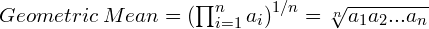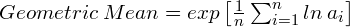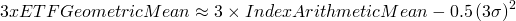Warning: "continue" targeting switch is equivalent to "break". Did you mean to use "continue 2"? in /home/calcul9/public_html/wp-content/themes/suffusion/functions/media.php on line 580

Warning: "continue" targeting switch is equivalent to "break". Did you mean to use "continue 2"? in /home/calcul9/public_html/wp-content/themes/suffusion/functions/media.php on line 583

Warning: "continue" targeting switch is equivalent to "break". Did you mean to use "continue 2"? in /home/calcul9/public_html/wp-content/themes/suffusion/functions/media.php on line 586

Warning: "continue" targeting switch is equivalent to "break". Did you mean to use "continue 2"? in /home/calcul9/public_html/wp-content/themes/suffusion/functions/media.php on line 589

Warning: "continue" targeting switch is equivalent to "break". Did you mean to use "continue 2"? in /home/calcul9/public_html/wp-content/themes/suffusion/functions/media.php on line 592
A Close Look at the Math Behind Leveraged ETF Returns » The Calculating Investor

# A Close Look at the Math Behind Leveraged ETF Returns

Apr 292011

#### An Application of the Geometric and Arithmetic Means

Over the past several years there has been a proliferation of ETFs for every imaginable purpose.

One particularly interesting type of ETF is the leveraged ETF. These ETFs are designed to provide 2X or 3X the daily return of a target index such as the S&P500, and they do a reasonable job of achieving this goal.

For example, if the S&P500 is up 1% for the day, the 2X S&P500 fund will be up 2% and the 3X fund will be up 3%. If the S&P500 is down 0.5% for the day, then the 2X fund will be down 1% and the 3X fund will be down 1.5%. (Note that for simplicity I’m not considering the fees and implicit borrowing costs associated with leveraged funds in this post).

Based on this description, you might think that if the S&P500 is up 10% for the year, then the 2X S&P500 fund would be up 20% and the 3X S&P500 fund will be up 30%. However, this is not the case. In fact, under some conditions, the 2X and 3X leveraged funds may be up even less than the S&P500 index. How can this be? If the daily leveraged returns are 2X or 3X the returns of the target fund, then why won’t the annual returns be 2X or 3X the annual returns?

To understand why there is discrepancy between daily returns and long-term returns, we need to review the difference between the arithmetic and geometric means.

##### Arithmetic Mean

The arithmetic mean is what most of us think of when we hear the term “average”, and it is defined by this equation.##### Geometric Mean

The geometric mean is the nth root of the product of n numbers, and it is defined by this equation:The geometric mean can also be calculated using logarithms:The geometric mean only applies for positive numbers. So, when using investment returns, the geometric mean is calculated using the decimal multiplier equivalent values. For example, a 6% return is 1.06, and a -4% return is 0.96.

##### Relationship Between the Arithmetic and Geometric Means

The geometric mean is always less than the arithmetic mean unless every number in the series is identical. The AM-GM inequality states that for a series of non-negative numbers:For a large series of returns that is normally distributed, the geometric mean is approximately equal to the arithmetic mean minus half of the variance.The intuition behind this difference can be seen the following example. Consider two investments A and B which are held for two periods. Investment A earns 10% in the first period and -10% in the second period. Investment B earns 30% in the first period and -30% in the second period. For both of these investments, the arithmetic mean return is zero percent. However, if an investor invested $100 in each investment, then the final value would be:So, even though the arithmetic mean return for the two investments is the same, the realized final value depends on the volatility. ##### Which Mean Should We Use for Investment Returns? When thinking about investment returns, both the arithmetic mean and the geometric mean are useful. The arithmetic return is the most familiar measure of the mean, and it is also the most commonly used metric in many statistical models such as the CAPM. However, it is not correct to compound the arithmetic mean to calculate the return realized over multiple periods. Whenever we are compounding returns, the geometric mean must be used. For example, if, over a ten year period, our arithmetic mean for the annual return was 10%, and the geometric mean over the same period was 8%, then the correct calculation of the current account value for an investor who invested$1000 at the beginning of the 10 year period would be:It would be incorrect, and would substantially overstate the final value, if we used the arithmetic mean of 10% in this calculation.

##### Application to Leveraged ETFs

With leveraged ETFs, an investor earns 2X or 3X the daily returns of the target index (less fees and borrowing costs), so the daily arithmetic mean of the 2X ETF is 2X the daily arithmetic mean of the target index, and the daily arithmetic mean of the 3X ETF is 3X the daily arithmetic mean of the target index.

However, since the daily variance is also increased, the daily geometric means do not go up by the same 2X or 3X factor.   If we use our approximation equation from above, we can estimate the geometric return for the target index and for the 2X and 3X leveraged ETFs.

Geometric Mean Return of Target Index:Geometric Mean Return of 2X Leveraged Fund:Geometric Mean Return of 3X Leveraged Fund:##### Empirical Data vs. Theoretical Estimates

Since our equation for estimating the geometric mean from the arithmetic mean and standard deviation is only an approximation (and our daily return data is a “fat tailed” distribution), it is a good idea to check the approximation equation against the empirical data.

In the plot below, I’ve used the actual daily returns of the U.S. market (based on the CRSP daily market return data) from 1964 through 2010.  For each year in the sample, I’ve calculated the daily arithmetic and geometric means, and I’ve also calculated the geometric and arithmetic means after doubling and tripling the daily returns.

The individual points on the plot show the difference between the geometric and arithmetic means vs. the daily standard deviation over each year.  The lines in the plot are based on the approximation equation.  Note that the curves match up very well with the empirical data and validate our approximation equation.Interestingly, the empirical data points that show a large error relative to our approximation equation are based on the return averages for 1987…which, of course, had one huge down day (October 19th or Black Monday) which was many standard deviations from the mean daily return.

Since the difference between the actual arithmetic and geometric mean returns match up very well with the approximation equation, we can use this equation to generate a reasonable prediction of what will happen under various volatility scenarios.

The arithmetic mean of the daily return over the full range of our data (1964-2010) is about 0.04% per day.  The plot below shows how the geometric means vary with standard deviation for this “typical” level of daily return.This plot shows that although the leveraged ETFs can outperform under some scenarios, they will do relatively poorly when volatility is high.  The crossover points will occur at lower volatilities in years when the daily returns have an arithmetic mean of less than 0.04%, and they will occur at higher volatilities in years when the arithmetic mean is higher.  Of course, the leveraged funds will always underperform for years with negative arithmetic mean.  Also, if we consider the borrowing costs, the leveraged ETFs will do even worse.

##### Conclusion

As the final graph shows, leveraged ETFs have some undesirable characteristics which investors should take the time to understand before investing in these funds.  It is a common misconception that these funds will return 2X or 3X the index returns over the long term, and, as the analysis shows, they are certain to fall short of this level of return.  In fact, there is a strong possibility that they will return even less than the the underlying target index.

If you feel you are capable of predicting short term market moves (good luck!), these funds may be an appropriate tool.  However, they are probably not a good choice for the long term investor.

Data:

Daily CRSP market return data can be found at the Kenneth French data library.

Code:

This R code was used to generate the plot comparing the historical data to the approximation equation:

# Download Daily Return Data

dret <- ff_daily[,2] + ff_daily[,5]

# Compute stats for full sample
darith <- mean(dret)
dstd <- sd(dret)

# Convert "date" column to year only for easy sorting
years <- trunc(ff_daily[,1]/10000)

# bind years variable to daily return variable
data <- cbind(years,dret)

# Init variables
d1x_a <- NULL
d1x_sd <- NULL
d1x_g <- NULL
d2x_a <- NULL
d2x_sd <- NULL
d2x_g <- NULL
d3x_a <- NULL
d3x_sd <- NULL
d3x_g <- NULL

# Calculate arithmetic and geometric returns for every year from 1964-2010
for(i in 1:47) {
returns <- subset(data,years==i+1963)
d1x_a[i] <- mean(returns[,2])
d1x_sd[i] <- sd(returns[,2])
d1x_g[i] <- 100*(exp(mean(log(returns[,2]/100+1)))-1)

d2x_a[i] <- mean(2*returns[,2])
d2x_sd[i] <- sd(2*returns[,2])
d2x_g[i] <- 100*(exp(mean(log(2*returns[,2]/100+1)))-1)

d3x_a[i] <- mean(3*returns[,2])
d3x_sd[i] <- sd(3*returns[,2])
d3x_g[i] <- 100*(exp(mean(log(3*returns[,2]/100+1)))-1)
}

# Calculate actual mean differences (geometric mean - arithmetic mean)
rdiff_1x <- d1x_g - d1x_a
rdiff_2x <- d2x_g - d2x_a
rdiff_3x <- d3x_g - d3x_a

# Calculate Theoretical Returns using approximation
rth_1 <- -50*((sort(d1x_sd)/100)^2)
rth_2 <- -50*((sort(d2x_sd)/100)^2)
rth_3 <- -50*((sort(d3x_sd)/100)^2)

# Plot Actual and Theoretical Data
dev.new(width=12,height=8)
rds <- cbind(rdiff_1x,rdiff_2x,rdiff_3x)
matplot(d1x_sd,rds,xlab="",ylab="",type="p",pch=c(1,2,3),lwd=c(2,2,2),cex.axis=1.3)
legend(0.35,-0.1,c("S&P 1X Actual","S&P 2X Actual","S&P 3X Actual", "S&P 1X Theoretical","S&P 2X Theoretical", "S&P 3X Theoretical"),col=c(1,2,3,1,2,3),pch=c(1,2,3,NA,NA,NA),lty=c(0,0,0,1,1,1),lwd=c(0,0,0,3,3,3),cex=1.75)
title("Return Difference vs. Standard Deviation",xlab="Daily Standard Deviation (%)",ylab="Geometric Mean - Arithmetic Mean (%)",cex.main="2.25",cex.lab="1.75")
lines(sort(d1x_sd),rth_1,lwd=2,col=1)
lines(sort(d1x_sd),rth_2,lwd=2,col=2)
lines(sort(d1x_sd),rth_3,lwd=2,col=3)


This R code was used to generate the second plot based on the approximation equation and the long term arithmetic average:


# Application Leveraged ETFs

# Set daily arithmetic mean to 0.04%
dailymean <- 0.0004

# Create a vector of standard deviations
stds <- 0:10 * 0.0025

# Calculate Geomean estimate using arithmean and standard deviations
sp1x <- dailymean - 0.5*stds^2
sp2x <- 2*dailymean - 0.5*(2*stds)^2
sp3x <- 3*dailymean - 0.5*(3*stds)^2

# Bind series and plot
means <- cbind(sp1x,sp2x,sp3x)
dev.new(width=12,height=8)
matplot(100*stds,100*means,type="l",xlab="",ylab="",lwd=c(3,3,3),cex.axis=1.3)
legend(1.75,0.12,c("S&P 1X","S&P 2X","S&P 3X"),lwd=c(3,3,3),col=c(1,2,3),cex=1.75)
title("Geometric Return vs. Standard Deviation",xlab="Daily Standard Deviation (%)",ylab="Daily Geometric Return (%)",cex.main="2.25",cex.lab="1.75")


### 7 Responses to “A Close Look at the Math Behind Leveraged ETF Returns”

1.Excellent analysis! I’ll refer to the math in a post on FAS-FAZ relationship.

•Hi Jev, Thanks for visiting my site. Your Quantum Blog site looks very interesting as well. I’ll try out some of the Matlab code.

2.This is really excellent analysis. Have you considered how leveraged inverse ETFs perform in relation to volatility?

If you follow Chang and Mandhavan’s (2009) analysis it would suggest that a -3x ETF has a higher expected decay than a 3x ETF as their exponential decay expression has a term -(x^2-x)*sigma^2.

3.The triple bull S&P 500 (UPRO) is up 159.1% from inception (25 Jun 09) to 9 Feb 2012. Over the same period, the S&P 500 is up 46.9%. Therefore the triple bull has returned considerably more than 3 times the S&P 500 price return. I believe this is because the effective leverage rises in a bull market, magnifying the returns. The UPRO significantly underperformed a perfect triple (exp(ln(s&p)*3)), but if you were bullish, you were very happy with this return. I know I am.

Note this leveraging factor works both ways. The UPRO significantly underperformed the S&P*3 during last years selloff.

To mimic a true triple leveraged investment, you would continually sell some upro over a bull market otherwise your original capital investment would become too highly leveraged. As you gradually withdrew funds in a bull market, your original capital would be returned and therefore not at risk, while you would continue to participate in the upside. When a big selloff occurs, you would buy more upro at the lower price with the cash you have withdrawn and play the game again. A very interesting option to consider.

•Oops, the upro is based on total return, not price. Bloomberg adjusts upro price history for this payout. So the correct comparison is with the S&P 500 total return index. The cumulative total return of the s&p 500 (25 jun 2009 to 9 feb 2012) is 55.04%. The cumulative total return of holding upro over the same time frame is 159.11%. Three times the S&P return is 165.12%, so the upro lagged the target by 6% or about 2% a year, corresponding to ‘fees and expenses’. The choice of time interval is important. If the market is down or only up modestly over the given interval, the upro will more significantly lag the target of 3 times the S&P 500 total return.

4. […] […]

5.Hi, I know this is an old post but, it is possible to estimate the time decay factor in an intraday basis? Thanks